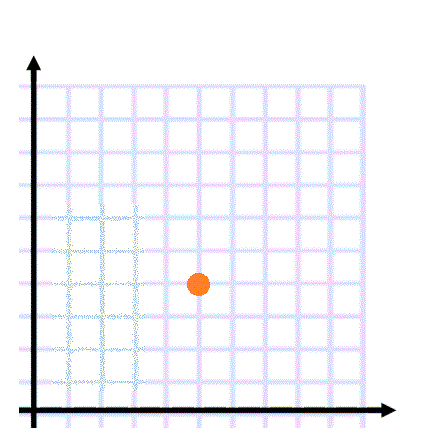# Trig Functions

Directions: Using the digits 1 to 9 at most one time each, fill in the empty blanks so that you create a triangle whose Cos Θ = √2/2: (5, 4), (__,__) and (__,__).### Hint

The cosine is formed by the relationships of what two sides of a triangle?
What unit triangle is needed to create a Cos Θ = √2/2?

There are many possible solutions, one is (6,2) and (8,3)

Source: Bryan Anderson

яндекс

## Period of Trig Function 3

Directions: Using the digits 0 to 9, at most one time, fill in the blanks …

### One comment

1.One possible solution is (8,3) and (6,2)